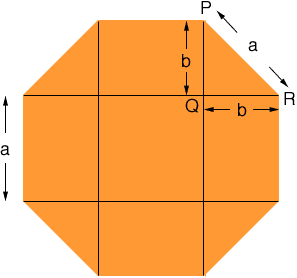Quandaries and Queries i need to find the area of an octagon with each side measuring 1 foot I am a student in seventh grade (middle school) Thank you, Jeremy Hi Jeremy, I assume your octagon is a regular octagon. Any figure in the plane that has straight line segments as its sides can be cut up into non overlapping triangular, square and rectangular pieces. If you can find the areas of the pieces you can add them to get the area of the figure. One way to cut up a regular octagon with side length a is illustrated in the diagram below.The octagon is cut into one square of area a2 square units four rectangles, each of area ab square units four triangles, each of area 1/2 bb square units. In your octagon a is 1 foot so all that remains is to find b. Triangle PQR is a right triangle so, by Pythagoras' Theorem a2 = b2 + b2 This equation allows you to find b and hence calculate the area of the octagon. Penny Go to Math Central